Select Page

# Motor Control

h

\$

\$

\$

\$

\$

\$

\$

## 1.1 Introduction

Electrical machines lab is one of the fundamental lab for the electrical engineering stream. The conventional machines laboratory courses can teach only the motor functionalities and drive concepts like induction motors and DC motors. During the lab session, the students will vary the voltage and current, using the autotransformer and rheostat to control the speed and load test of the motor. While it is important to teach the conventional motor setups, it is also equally important to give an introductory experience to the motor drives setup, their pulse control & commutation, VFDs, etc. It will enable the students to stay in line with the present industry requirements.

We offer Data Acquisition & Control systems that can be interfaced with external drivers & motors from industry vendors like Rockwell, Siemens, Kirloskar etc.

The compact RIO (cRIO) based NI embedded control platform gives an option to implement the PWM techniques for the inverters, DC-DC converters, 6 step commutation techniques, special machine control etc. The LabVIEW based graphical programming environment provides an intuitive method for students to acquire, visualize and process the signal from the experiment setup. With the help of these hardware setup, student can get the feel of motors and drives used in the industry.

## 1.2 Benefits of RIO hardware platform

1. Rich visualization of voltage, current, speed and other signals

2. Students can observe transients, spikes, phase differences, harmonics, etc. while doing the experiments

3. Open platform for Data acquisition & Control

4. In addition to learning the conventional motor concepts, the students can learn concepts related to PWM, SPWM, SVPWM, 6 step control, PID Control, etc.

5. Vrms, Irms, Apparent power, real power, power factor, harmonics, etc. can be computed by students in LabVIEW, rather than directly reading from the instruments.

6. Simple & powerful graphical programming approach using LabVIEW

7. The same LabVIEW, DAQ and cRIO are also used in industry for implementing test, measurement and control systems.

## 1.3 Required tools

We require following hardware and software to automate the electrical machines setup.

## Hardware

1.  NI LabVIEW
2.  NI Real-Time Module
3.  NI FPGA Toolkit
4.  NI FPGA Compiler Farm
6.  NI CompactRIO Device Driver
7.  VI Package Manager
8.  NI Floating point FPGA Library
9.  PE Libraries for FPGA
 CompactRIO sbRIO Voltage Measurement NI 9225 / NI 9242 / NI 9244 Not Available Current Measurement NI 9227 / NI 9246 / NI 9247 Speed Measurement (Proximity/Encoder) NI 9401 / NI 9402 / NI 9411 NI 9683 / NI 9684 PWM Generation NI 9401 / NI 9474 Analog Input NI 9202 / NI 9215 / NI 9220/ NI 9223 Controller NI cRIO 904x / NI cRIO 905x / NI cRIO 903x / NI cRIO 906x sbRIO 96xx with RMC Converter for Induction motor / BLDC Motor / PMSM Motor 3 Leg Converter with builtin singal conditioning DC Motor DC DC Converter SRM Motor SRM Motor Controller

All examples in ths course are prepared based up the NI 9054 cRIO hardware with NI 9220 analog input module and 9401 digital input/output module. This configuration can be replaced with any other RIO hardware architecture by considering the following parameters.

Controller: Processing power + FPGA size + RAM + Number of slots + Network connectivity

Analog input module: Number of channels + Sampling rate + Resolution + Voltage range

Digital input/output module: Number of channels + Update rate + Voltage level

## 1.4 PE libraries for FPGA

This library contains the basic functions required for the power electronics and motor control applications. It contains three basic sections.

1.  Waveforms
2.  Time
3.  PWM

## 1.4.1 Waveforms

One common method to generate the repetitive waveforms with high degree of frequency and phase control is Direct Digital Synesis (DDS) method. The waveforms are having better relative frequency control as well as precise phase control between multiple waveforms. All the waveforms are configured using express VI with desired signal frequency and constant amplitude (+/-1). User can dynamically change the signal frequency using the Frequency (periods / ticks) node. DDS implementation requires three main hardware building blocks.

1. Sample Clock

2. Phase accumulator

3. Lookup table

The block diagram of the Direct Digital Synthesis is given below.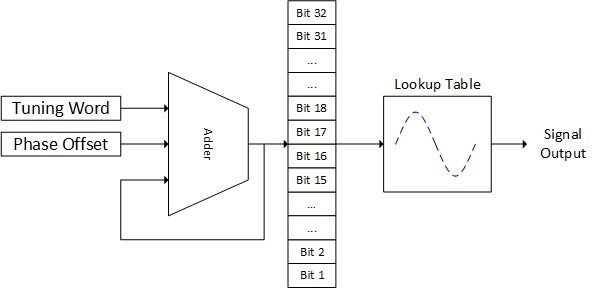Block diagram of the Direct Digital Systhesis Architecture

Sample clock

The sample, or reference, clock is used to create the frequency tuning word, update the phase accumulator value, and generate the output signal. The sample clock determines when a sample is generated, but it does not directly determine the frequency of the output signal.

Phase accumulator

To create the signal at a precise frequency, the phase accumulator uses three general components.

1. The phase accumulator uses the tuning word to specify the frequency of the signal. The tuning word is a 24 to 48-bit digital word, that specifies how many samples to jump in the waveform memory.

2. The adder takes the tuning word and sums it to the phase register remainder. The new digital value is output to the phase register.

3. The final component of the phase accumulator, the phase register, takes the new digital word and use it to specify the memory address of the next sample point to the output in the lookup table. The phase register takes the remaining most significant bits not used in the lookup table memory address and provides them back to the adder to ensure frequency precision over time.

Lookup table.

The output of the phase register only looks like a digital ramp as the memory address increases over time, which is changing at the rate specified by the tuning word. Therefore, to output the wanted waveform, the output of the phase register points to the needed waveform samples address in the lookup table. The lookup table then provides the digital word at the provided memory address, which is the digital word of the correct amplitude and phase for the output waveform.

Waveform palette

The waveform palette contains the following functions

1. Sine waveform

2. Triangle waveform

3. Sawtooth waveform

4. Third harmonic waveform

5. Three phase sine waveforms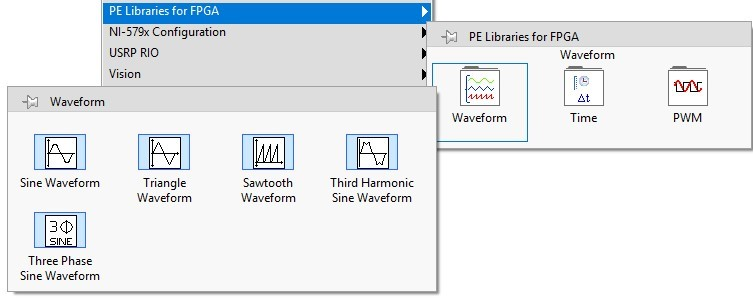The express VI helps to configure the signal frequency easily in the waveform configuration dialog box. To change the signal frequency dynamically, user can use the input available for the signal generation node. The frequency of the signal is specified as frequency (periods/ ticks).

Frequency (periods/ticks) =Signal Frequency (Hz) / Base Clock Frequency(Hz)

The signal phase is specified as phase offset.

Phase offset= Signal Phase (Degree) / 360

All the waveform generation functions are compatible with Single Cycled Timed Loop (SCTL).

For example, the sine waveform configuration window given below.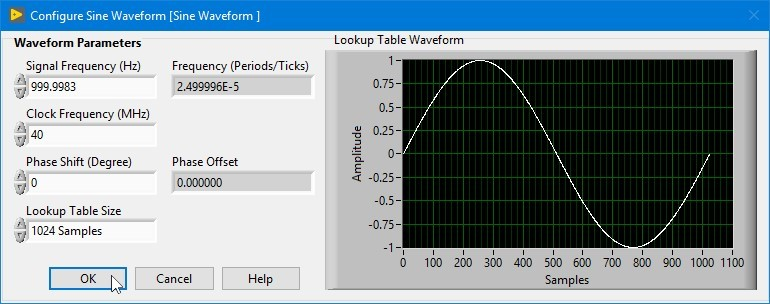The Sine waveform configuration window will help the user to direct specify the signal frequency (in Hz) and phase shift (in degree). This configuration automatically tune the desired frequency to the closed available frequency. Using Lookup Table Size, user can select the desired number of samples in the reference reference waveform.Using the above mentioned formula, user can programmatically update the frequency(periods/ticks) and Phase offset. This will override the values specified in the configuration window.

## 1.4.2 Time

All the functions are executed based on the FPGA Clock. The waveform generation and PWM functions runs based on the sample clock. This palette provides the basic time related functions. The VIs available in the Time sub palette is shown in the figure 3.3.

1. Loop time in ticks

2. Rising delay (in iterations)

3. Zero crossing delay (in iterations)

4. Speed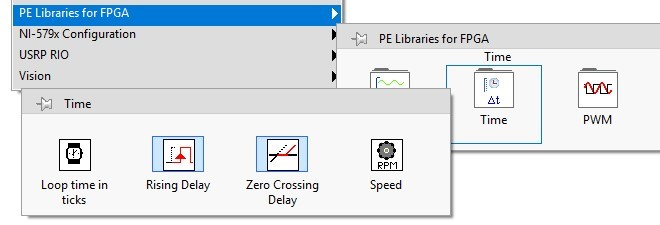Loop time in ticks

This block provides the loop time in tick counts (or machine cycles). This block provides the time required to complete one iteration in ticks independent of the base clock.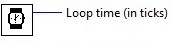Rising delay (in iteration)

This block helps us to provide the dead time for the PWM. Whenever the Boolean value changes from False to True state, we can introduce rising delay based on the loop time. During this period, the Boolean output remains in the False state.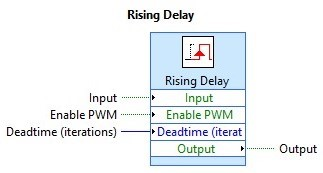For example, the loop takes 10 ticks to complete one iteration in 40MHz clock. The rising delay (in iteration) is specified as 5. The resultant delay time is calculated as given below.

Base Clock = 40MHz

1 tick = 1 / Base clock = 1 / 40MHz = 25nS

Loop time (in ticks) = 10 Loop time (in nS) = 10 x 25 = 250nS

Rising delay (in iteration) = 5 Rising delay (in nS) = 5 x 250ns = 1250nS or 1.25uS

It will provide 1.25uS of deadtime to the Boolean output.

The rising delay (in iteration) is calculated as given below,

Rising delay(in iteration)=Total Delay(in second) / ( Loop time x 1 /Base clock)

Zero crossing delay (in iteration)

This Express VI introduces delay time for the signal when it cross zero in rising edge. While crossing the zero in rising edge (negative to positive), user can introduce the delay for the signal, and it remains zero during the delay period. The zero-crossing delay is calculated as given formula.

Zero-crossing delay(in iteration)=Total Delay(in second)/ ( Loop time x 1 / Baseclock)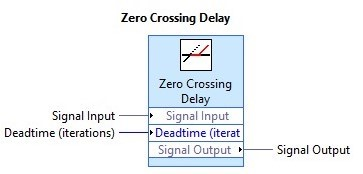Speed

This VI calculate the speed of the motor using input pulses. Normally proximity or hall sensor pulses are used to calculate the speed of the motor.

Base Clock: FPGA Base clock Frequency (in Hz). The default value is 40MHz.

Pulse per revolution: Number of pulses generated per revolution. The default value is 1.

Cycles to Filter: Number of Clock cycles to filter the noise signal in the proximity input. If noise signals are present in the hall sensor feedback, increase the value gradually up to 100 cycles. The default value is 0.## 1.4.3 PWM

This palette provides the functions to generate the various PWM techniques to control the motor. The VIs available in the PWM sub palette is given below.SPWM

This block can generate the Sine PWM signal for controlling the single-phase inverter. Sine waveform (reference) (Vref) is compared with a high frequency triangle waveform (carrier) (Vc) to generate the switching signals for the inverter.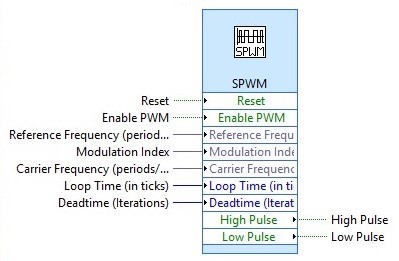The inverter output is controlled by the following parameters:

1. PWM frequency is the same as the triangle waveform frequency (Fc)

2. Amplitude is controlled by amplitude of the sine waveform (Vref)

3. Fundamental frequency of the output is controlled by sine frequency (Fs)

Modulation index (m) is defined as,

m= Vref  / Vc = Amplitude of the Sine (Vref) / Amplitude of the triangle(Vc)

Deadtime (in iterations) = Deadtime (in second) / ( Loop time (in ticks) x 1 / Base Clock)

For example, the SPWM configuration window is given below.User can specify the reference signal (sine) frequency and carrier frequency (Triangle) in SPWM configuration window. The sine waveform amplitude is controlled using modulation index. We can specify the dead time as number of iterations. Also we can generate the SPWM pules in following two methods.

1. Bipolar

2. Unipolar

Bipolar

In Bipolar SPWM, the upper and lower switches in the same leg work in complementary to each other.

When Vref > Vc, G1 is ON

Vref < Vc, G2 is ON.

Deadtime is introduced in the Boolean output as rising delay (in iteration) for both the gate pulses.

Unipolar

The unipolar SPWM requires two reference signals (Vref and -Vref) which are in the same magnitude and frequency but 180 degree out of phase. Both the reference sine waveforms are compared with carrier triangle waveform and pulses are generated.

When Vref > 0 and Vref > Vc, G1 is ON

When -Vref > 0 and -Vref >Vc, G2 is ON.

The end user can change the triangle waveform amplitude between ±1 (unipolar – I) or 0 to 1 (Unipolar – II) depending up on the application.

Deadtime is introduced in the form of zero-crossing delay (in iteration) for the reference sine waveforms (Vref and -Vref).

Three Phase SPWM

This block generates the Three Phase Sine PWM for the three-leg inverter. The user having the option to control the reference signal amplitude, frequency and PWM carrier frequency. It works similar to the SPWM technique for three phase waveforms.PWM

This function used to generate the constant pulses. The duty cycle (Vref) is compared with the sawtooth waveform (Vc) and output pulses are generated.

When Vref > Vc, G1 is ON

Vref < Vc, G1 is OFFThis function is compatible with Single Cycle Timed Loop (SCTL).

BLDC Motor control

This is used to control the BLDC Motor operation based on the hall sensor. PWM pulses are generated based on the duty cycle (Vref) and sawtooth waveform (Vc). Based on the BLDC Motor position, the switching sequence is controlled.The 6-step commutation or 120 degree trapezoidal control is characterized by a two-phase ON operation to control the three phase inverter. In this method, torque production follows the principle that current should flow in two of the three phases at the same time and that the angle between the stator magnetic field and the rotor flux is kept close to 90 degrees to get the maximum generated torque. The BLDC Motor phase voltages, current and hall sensor waveforms with respect to the rotor electrical angle is given below.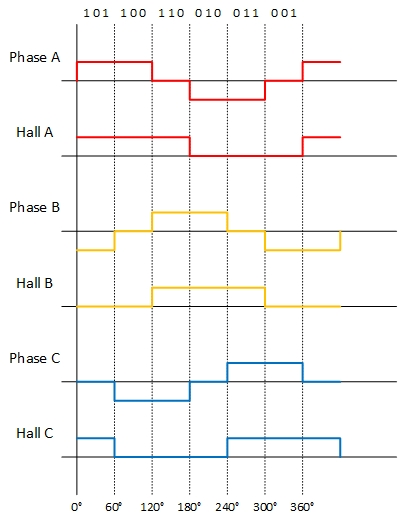The BLDC Motor having 3 hall sensors placed 120 degree from each other. This provides the required digital signals for the controller to dermine the rotor position in intervals of 60 electrical degrees. In 120 degree hall placement, it never generates the code in which all the hall sensors signal are high or low simultaneously. The binary codes 111 or 000 are invalid and this allows for an easier fault detection mechanism.

The six state commutable table for the BLDC is motor is given below:

 Hall Sensor Motor Phase Hall A Hall B Hall C Phase A Phase B Phase C 1 1 0 OFF + – 0 1 0 – + OFF 0 1 1 – OFF + 0 0 1 OFF – + 1 0 1 + – OFF 1 0 0 + OFF –

The + sign in the table denotes that back emf in the particular phase is positive and a positive current must be injected in phase (top switch ON), and – sign denotes that back emf is negative and negative current must be injected in the phase (bottom switch ON).

PMSM Motor

This is used to control the PMSM Motor operation in 120 mode conduction or six step commutation based on the hall sensor. PWM pulses are generated based on the duty cycle (Vref) and sawtooth waveform (Vc). The PMSM motor 120 mode operation is similar to BLDC Motor.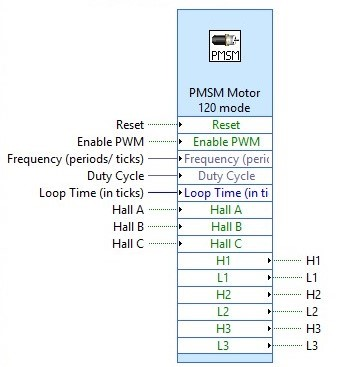SRM Motor

This SRM motor control function is used to control the 4 phase SRM Motor (8 stator poles and 6 rotor poles) using (n+1) switches and (n+1) diode bridge configuration (where n is the number of phase winding). Based on the rotor position measured using proximity sensor, the output pulses are controlled.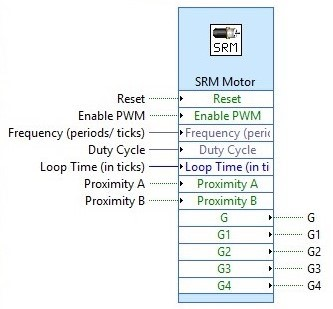Proximity A need to be placed to get the high signal when the rotor align with the windings 1 & 2. Proximity B need to be placed to get the high signal when the rotor align with the windings 1 & 4.

error: Content is protected !!